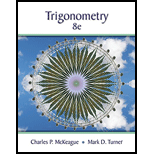Chapter 5.3, Problem 5PSTrigonometry (MindTap Course List)

8th Edition
Charles P. McKeague + 1 other
ISBN: 9781305652224

Solutions

Chapter
SectionTrigonometry (MindTap Course List)

8th Edition
Charles P. McKeague + 1 other
ISBN: 9781305652224
Textbook Problem

For Qustions 4 through 6, determine if the statement is true or false. cos 2 A = 2 cos A

To determine

To state:

If the given statement is TRUE or FALSE.

Explanation

Given information:

cos2A=2cosA

We need to choose some value of A and check whether the given statement is TRUE or FALSE. Using A=π6

 cos2A=2cosA cos2⋅π6=?2cosπ6 cos2⋅π36

Still sussing out bartleby?

Check out a sample textbook solution.

See a sample solution

The Solution to Your Study Problems

Bartleby provides explanations to thousands of textbook problems written by our experts, many with advanced degrees!

Get Started

Evaluate the integral by making the given substitution. cos2xdx,u=2x

Single Variable Calculus: Early Transcendentals, Volume I

In Exercises 75-98, perform the indicated operations and/or simplify each expression. 94. (x2 1)(2x2 x2(2x)

Applied Calculus for the Managerial, Life, and Social Sciences: A Brief Approach

Solve the equations in Exercises 112 for x (mentally, if possible). x1=cx+d(c1)

Finite Mathematics and Applied Calculus (MindTap Course List)

In Exercises 1-22, evaluate the given expression. C(5,0)

Finite Mathematics for the Managerial, Life, and Social Sciences

True or False: div curl F = 0.

Study Guide for Stewart's Multivariable Calculus, 8th